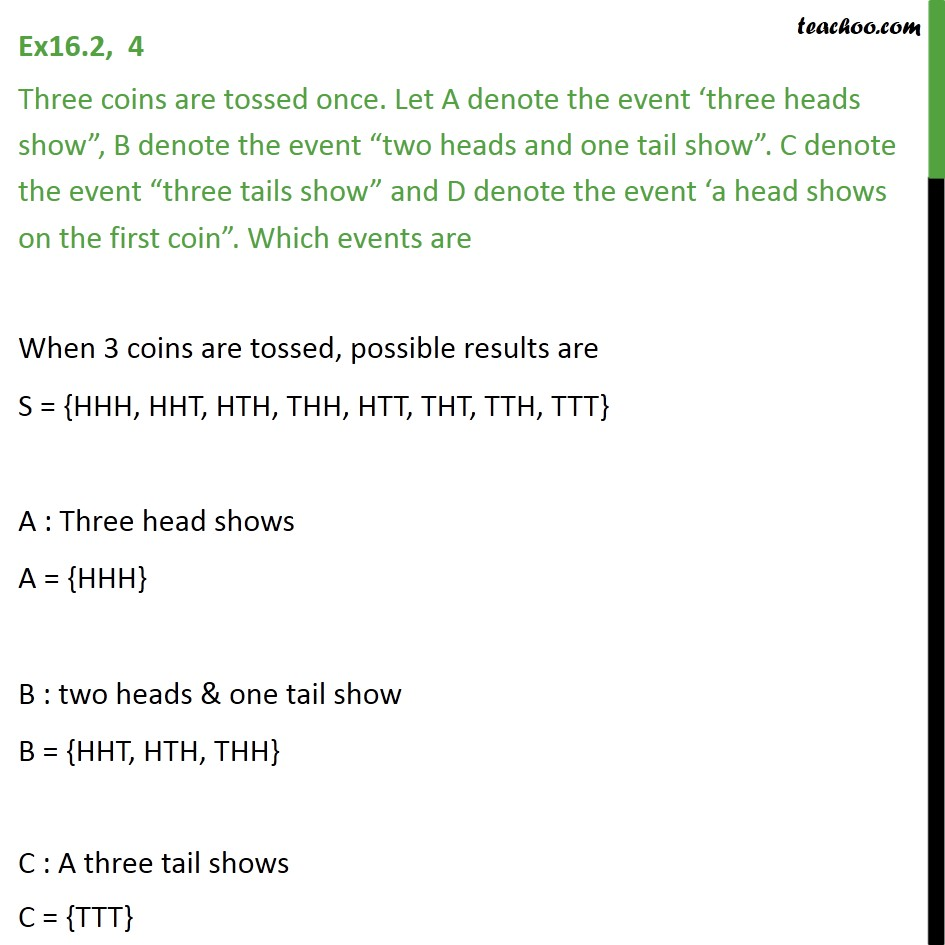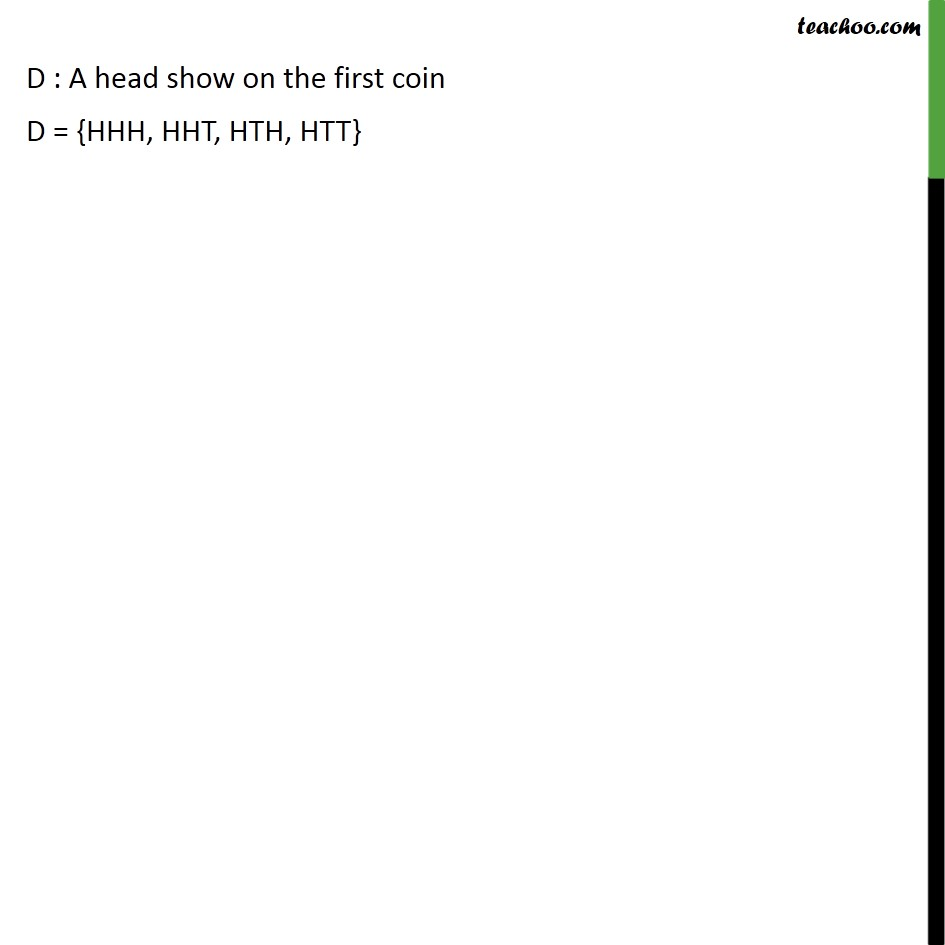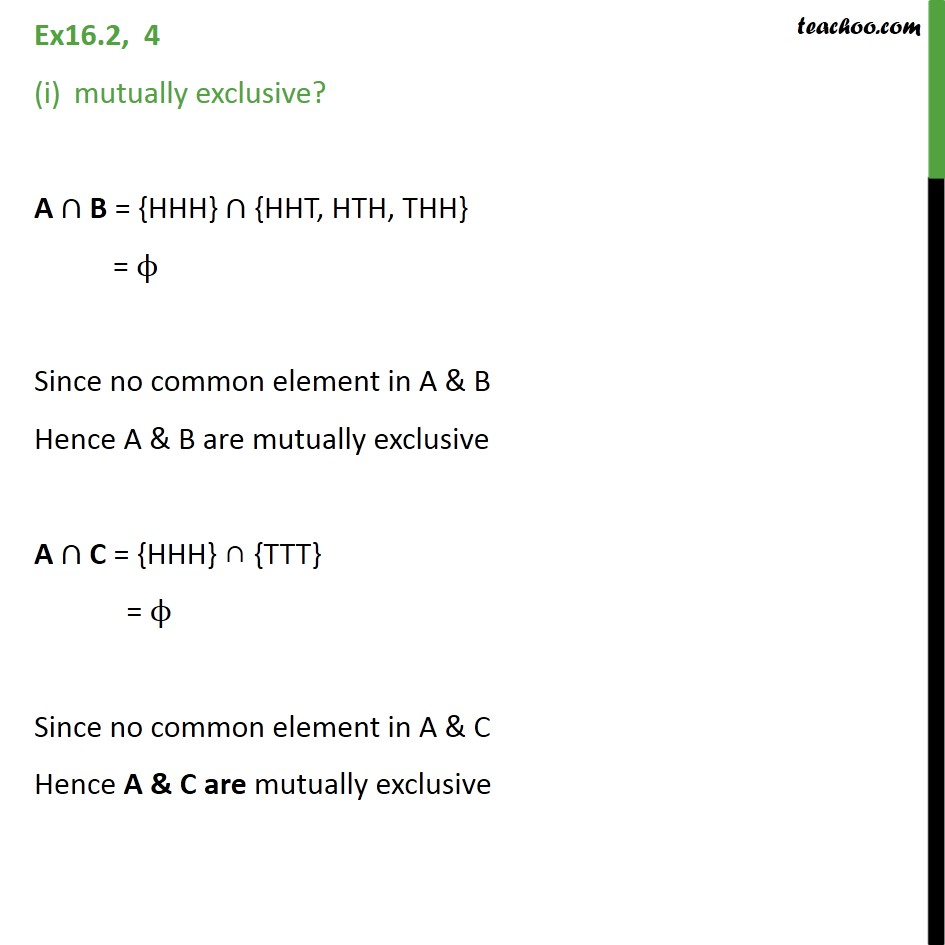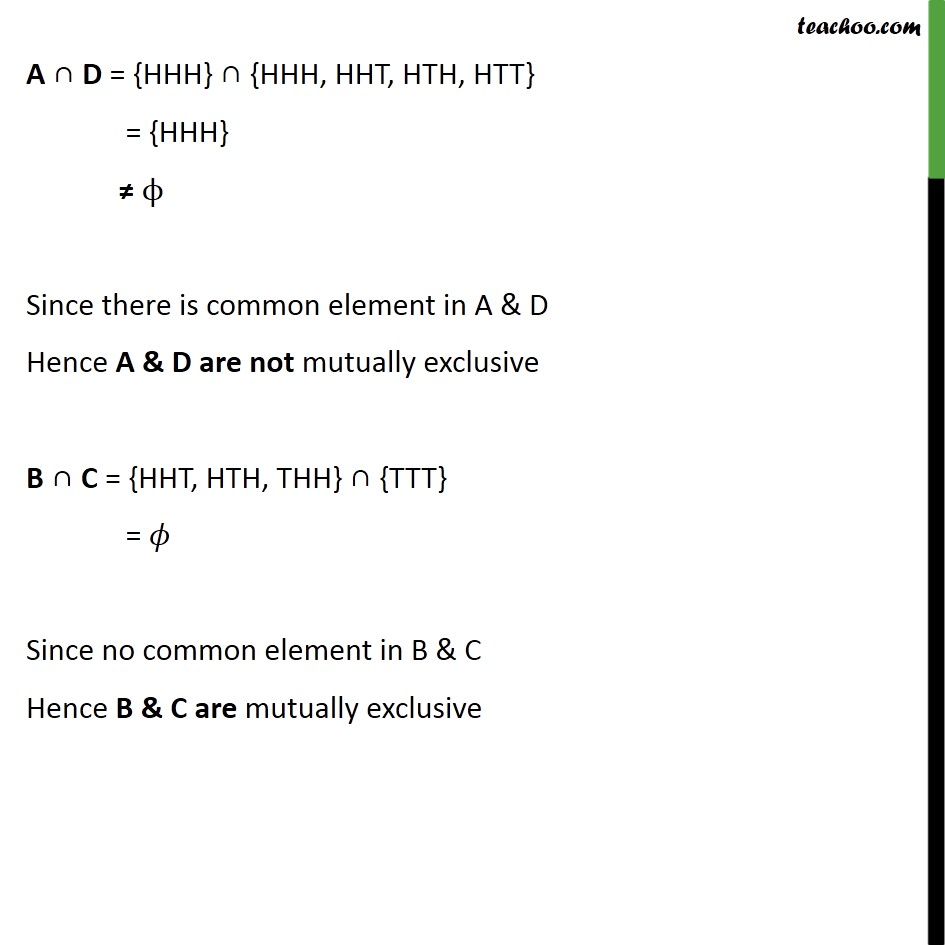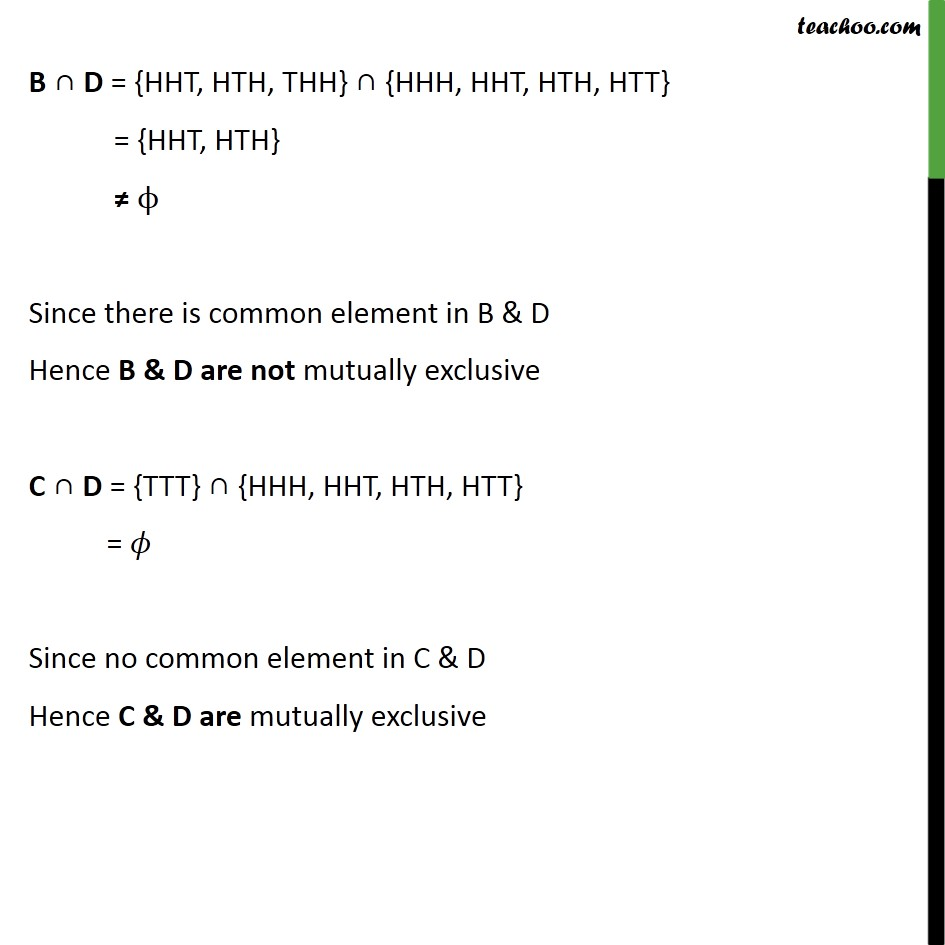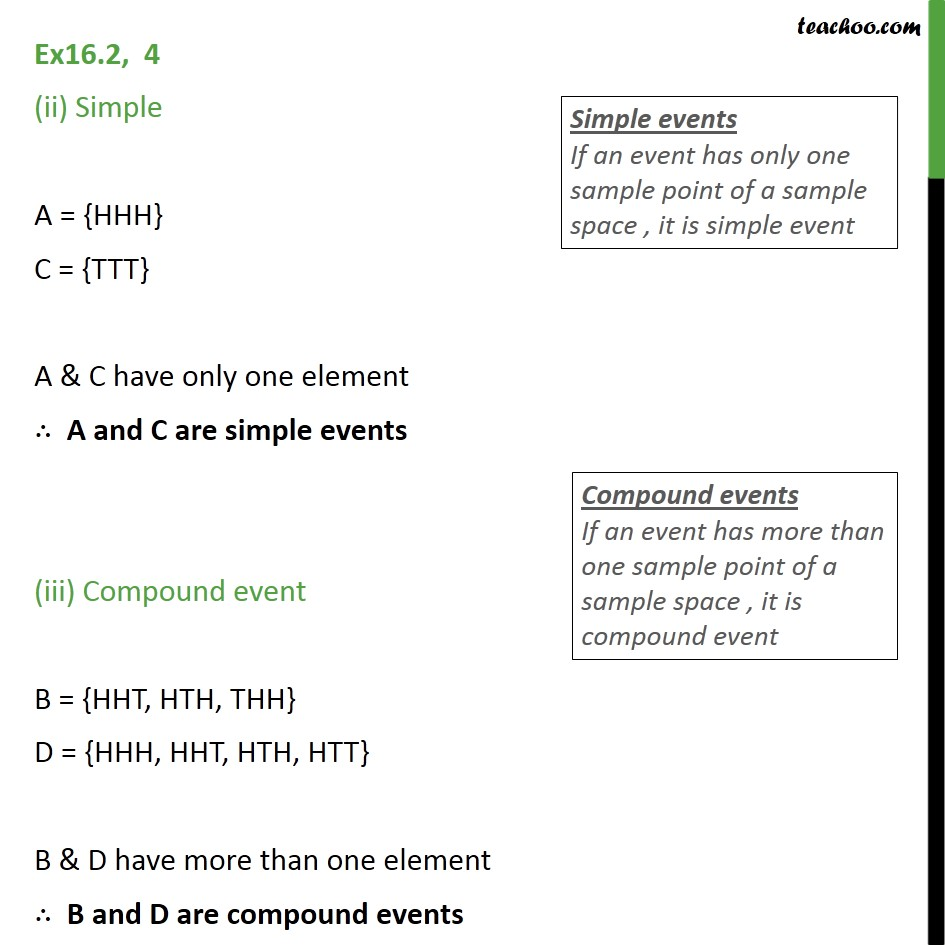1. Chapter 16 Class 11 Probability
2. Serial order wise
3. Ex 16.2

Transcript

Ex16.2, 4 Three coins are tossed once. Let A denote the event three heads show , B denote the event two heads and one tail show . C denote the event three tails show and D denote the event a head shows on the first coin . Which events are When 3 coins are tossed, possible results are S = {HHH, HHT, HTH, THH, HTT, THT, TTH, TTT} A : Three head shows A = {HHH} B : two heads & one tail show B = {HHT, HTH, THH} C : A three tail shows C = {TTT} D : A head show on the first coin D = {HHH, HHT, HTH, HTT} Ex16.2, 4 mutually exclusive? A B = {HHH} {HHT, HTH, THH} = Since no common element in A & B Hence A & B are mutually exclusive A C = {HHH} {TTT} = Since no common element in A & C Hence A & C are mutually exclusive A D = {HHH} {HHH, HHT, HTH, HTT} = {HHH} Since there is common element in A & D Hence A & D are not mutually exclusive B C = {HHT, HTH, THH} {TTT} = Since no common element in B & C Hence B & C are mutually exclusive B D = {HHT, HTH, THH} {HHH, HHT, HTH, HTT} = {HHT, HTH} Since there is common element in B & D Hence B & D are not mutually exclusive C D = {TTT} {HHH, HHT, HTH, HTT} = Since no common element in C & D Hence C & D are mutually exclusive Ex16.2, 4 (ii) Simple A = {HHH} C = {TTT} A & C have only one element A and C are simple events (iii) Compound event B = {HHT, HTH, THH} D = {HHH, HHT, HTH, HTT} B & D have more than one element B and D are compound events

Ex 16.2